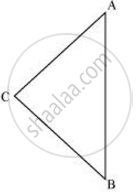# ABC is an isosceles triangle with AC = BC. If AB^2 = 2AC^2, prove that ABC is a right triangle. - Mathematics

ABC is an isosceles triangle with AC = BC. If AB2 = 2AC2, prove that ABC is a right triangle.

#### SolutionGiven that

AB2 = 2AC2

⇒ AB2 = AC2 + AC2

⇒ AB2 = AC2 + BC2 (As AC = BC)

The triangle is satisfying the pythagoras theorem

Therefore the given triangle is a right angle trangle

Concept: Right-angled Triangles and Pythagoras Property
Is there an error in this question or solution?

#### APPEARS IN

NCERT Class 10 Maths
Chapter 6 Triangles
Exercise 6.5 | Q 5 | Page 150